##### Proposing an Image Enhancement Algorithm Using CNN for Applications of Face Recognition System

Phat Nguyen Huu, Loc Hoang Bao, Hoang Lai The

Journal of Advances in Mathematics and Computer Science, Page 1-14
DOI: 10.9734/jamcs/2019/v34i630230

Many researches have been going on since last two decades for object recognition, shape matching, and pattern recognition in the field of computer vision. Face recognition is one of the important issues in object recognition and computer vision. Many face image datasets, related competitions, and evaluation programs have encouraged innovation, producing more powerful facial recognition technology with promising results. In recent years, we have witnessed tremendous improvements in face recognition performance from complex deep neural network architectures trained on millions of face images. Face recognition is the most important biometric and stills many challenges such as pose variation, illumination variation, etc. In order to achieve the desired performance when deploying in reality, the methods depend on many factors. One of the main factors is quality of input image. Therefore, facial recognition systems is installed outdoors which are always affected by extreme weather events such as haze, fog. The existence of haze dramatically degrades the visibility of outdoor images captured in inclement weather and affects many high-level computer vision tasks such as detection and recognition system. In this paper, we propose a preprocessing method to remove haze from input images that enhances their quality to improve effectiveness and recognition rate for face identification based on Convolutional Neural Network (CNN) based on the available datasets and our self-built data. To perform the proposed method for outdoor face recognition system, we have improved the system accuracy from 90.53% to 98.14%. The results show that the proposed method improves the quality of the image with other traditional methods.

##### Adopting Tolerance Regions in Environmental Economics

Christos P. Kitsos, Thomas L. Toulias

Journal of Advances in Mathematics and Computer Science, Page 1-12
DOI: 10.9734/jamcs/2019/v34i630227

Uncertainty often lies when there is limited knowledge about the process one has to follow regarding the investigation of a real-world problem. In practice, uncertainty is related with the assumed estimation model of the physical problem, and mainly concerns the involved parameters. A typical example
can be an Environmental Economics system. There are many model specifications that estimate the so-called Benefit Area of such system. For the evaluation of the optimal level of pollution, we can adopt the corresponding tolerance region, and hence we can refer to this optimal level via future observations rather than some parameters estimation. Tolerance regions can be either classical or expected tolerance regions. The associated (four) Benefit Areas can be evaluated through a proposed tolerance region procedure, and not through the usual confidence interval/region approach. Therefore, four possible optimal levels of pollution can be obtained, as well as the corresponding tolerance region for the reduction pollution point.

##### Transverse Motions of Bernoulli-euler Beam Resting on Elastic Foundation and under Two Concentrated Moving Loads

Journal of Advances in Mathematics and Computer Science, Page 1-21
DOI: 10.9734/jamcs/2019/v34i630228

Aims/Objectives: The aim is to obtain a closed form solutions of single-dimensional structural element of continuously supported by an elastic foundation. Thereafter, we classify the effects of the space d connecting the loads on the relevant partial differential equations governing the motion of the structural members. The study also analysis circumstances under which resonance occur in the dynamical systems involving structural members.

Study Design: The single-dimensional structural element is a partial differential equation of order fourth order place on elastic Winkler foundation. The Bernoulli-Euler beam traversed by two moving loads.

Place and Duration of Study: Department of Mathematical Sciences, Adekunle Ajasin University P.M.B. 01, Akungba-Akoko, Nigeria, between May 2019 and September 2019.

Methodology: The principal equation of the single -dimensional beam model is governing by partial differential equation of the order four. For the single -dimensional beam problem, the solution techniques are based on the Fourier sine transformation. The governing partial differential equation of the order four was reduced to sequence of second order ordinary differential equations.

##### Modelling Factors Affecting Lung Capacity

Wanyonyi Maurice

Journal of Advances in Mathematics and Computer Science, Page 1-18
DOI: 10.9734/jamcs/2019/v34i630229

This study aims to evaluate modelling factors affecting lung capacity using linear Regression model. The study employed multiple regression models which were used to fit the factors affecting lung capacity. The factors affect lung capacity includes the following; age, gender, smoking and height. The objectives of the study were; fitting regression model on factors affecting lung capacity, determining the relationship between age and height with lung capacity. The study aim also includes predicting the value of lung capacity using the fitted model.

The data used in this study was a secondary source which was obtained from Marin . The dataset is publicly available on their website. The data had 725 observations. Since multiple linear regression model was employed in this study, the model was of the form;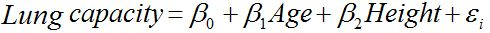Where;

Lung capacity is the dependent variable,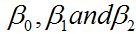are the coefficients (parameters) to be estimated,

Age and Height are the independent variables while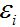is the random error component. The methods of parameter estimation discussed under this study include; maximum likelihood estimator and the least square estimator.

The data for this study were analyzed using SPSS and R software which are statistical software used for data analysis. From the analysis of variance table, a p-value of 0.00 was recorded which is less than alpha (alpha= 0.05). This implies that the overall model is significant.

From the model formulated, it was concluded that height and age greatly affect lung capacity. The model formulated can be used to predict the value of lung capacity provided the values of Age and Height are known. Also from the descriptive statistics, it is deduced that gender and smoking greatly affect lung capacity.

##### Determination of the Equilibrium Point According to a Temporal Model of Rehydration in a Population with Cholera in Logistic Growth

K. O. Jackob

Journal of Advances in Mathematics and Computer Science, Page 1-5
DOI: 10.9734/jamcs/2019/v34i630231

Cholera is an infection of the small intestine of humans caused by a gram-negative bacterium called Vibrio cholerae. It is spread through eating food or drinking water contaminated with faeces from an infected person. It causes rapid dehydration and general body imbalance, and can lead to death since untreated individuals suer severely from diarrhea and vomiting. Its dynamics involves multiple interaction between the human host, the pathogen and the environment which contributes to both human to human and indirect environment to human transmission pathways. Rehydration is critical in reducing cholera death. This has been studied by other scholars but they did not consider delay in rehydration on the spread of cholera. In this paper, I formulate a mathematical model based on system of ordinary differential equation with rehydration on the spread of cholera in a logistically growing population. The existence of the steady states and the basic reproduction number is established such that disease free equilibrium point exists. Determination of endemic equilibrium shows that the model has positive points. The findings will be signicant in the sense that timely rehydration should be done during cholera outbreak and will enable individuals with symptoms to seek immediate medical attention.

##### An Immersed Finite Element Method for Planar Elasticity Interface Problem

Angran Liu

Journal of Advances in Mathematics and Computer Science, Page 1-16
DOI: 10.9734/jamcs/2019/v34i630232

This paper presents the P1/CR immersed finite element (IFE) method to solve planar elasticity
interface problem. By adding some stabilisation terms on the edges of interface elements, the
stability of the discrete formulation and a priori error estimate in an energy norm are presented.
Finally, numerical examples are given to confirm our theoretical results.

##### Bayesian Optimization for Parameter of Discrete Weibull Regression

Journal of Advances in Mathematics and Computer Science, Page 1-13
DOI: 10.9734/jamcs/2019/v34i630233

This study aim at optimizing the parameter θ of Discrete Weibull (DW) regression obtained by maximizing the likelihood function. Also to examine the strength of three acquisition functions used in solving auxiliary optimization problem. The choice of Discrete Weibull regression model among other models for fitting count data is due to its robustness in fitting count data. Count data of hypertensive patients visits to the doctor was obtained at Medicare Clinics Ota, Nigeria, and was used for the analysis. First, parameter θ  and β  were obtained using Metropolis Hasting Monte Carlo Markov Chain (MCMC) algorithm. Then Bayesian optimization was used to optimize the parameter the likelihood function of DW regression, given β to examine what θ would be, and making the likelihood function of DW the objective function. Upper confidence bound (UCB), Expectation of Improvement (EI), and probability of Improvement (PI) were used as acquisition functions. Results showed that fitting Bayesian DW regression to the data, there is significant relationship between the response variable, β and the covariate. On implementing Bayesian optimization to obtain parameter new parameter θ of discrete Weibull regression using the known β, the results showed promising applicability of the technique to the model, and found that EI fits the data better relative to PI and UCB in terms of accuracy and speed.

##### Matrix Representation of Bi-Periodic Jacobsthal Sequence

Sukran Uygun, Evans Owusu

Journal of Advances in Mathematics and Computer Science, Page 1-12
DOI: 10.9734/jamcs/2019/v34i630234

In this paper, we bring into light the matrix representation of bi-periodic Jacobsthal sequence, which we shall call the bi-periodic Jacobsthal matrix sequence. We dene it as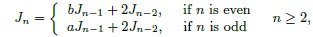with initial conditions J= I identity matrix,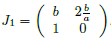. We obtained the nth general term of this new matrix sequence. By studying the properties of this new matrix sequence, the well-known Cassini or Simpson's formula was obtained. We then proceed to find its generating function as well as the Binet formula. Some new properties and two summation formulas for this new generalized matrix sequence were also given.